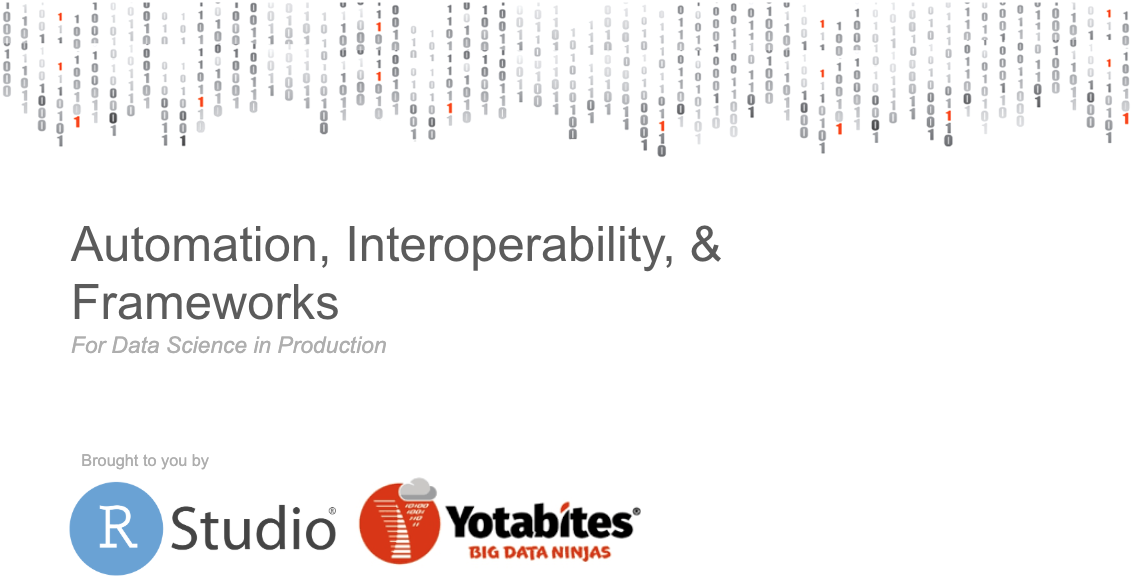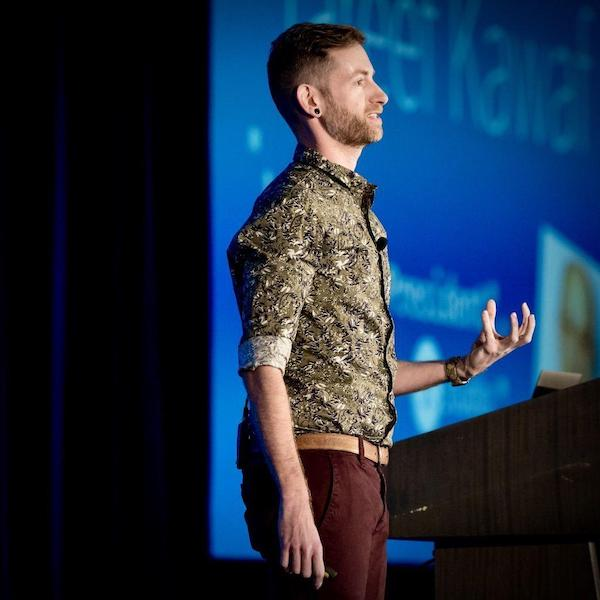# RStudio Beta 3 (v0.94)

2011-06-14 RStudio Team
RStudio Beta 3 (v0.94) is available for download today. The goal for this release was to refine and improve our core features based on the feedback we’ve gotten on our first two betas. Highlights of the new release include: Source editor enhancements — New editor features include brace/paren/quote matching, more intelligent cursor placement after newlines, function navigation, regex find and replace, run to/from the current line, and a command to re-run the last code region. Read more →

# RStudio Beta 2 (v0.93)

2011-04-11 RStudio TeamRStudio Beta 2 (v0.93) is available for download today. We’ve gotten incredibly helpful input from the R community and this release reflects a lot of that feedback. The release notes have the full details on what’s new. Some of the highlights include: Source Editor Enhancements Highlight all instances of selected text Insert spaces for tabs (soft-tabs) Customizable print margin line Selected line highlight Toggle line numbers on/off Read more →

# RStudio, new open-source IDE for R

2011-02-28 RStudio TeamRStudio is a new open-source IDE for R which we’re excited to announce the availability of today. RStudio has interesting features for both new and experienced R developers including code completion, execute from source, searchable history, and support for authoring Sweave documents. RStudio runs on all major desktop platforms (Windows, Mac OS X, Ubuntu, or Fedora) and can also run as a server which enables multiple users to access the IDE using a web browser. Read more →

2011-02-27 RStudio Team
We started the RStudio project because we were excited and inspired by R. The creators of R provided a flexible and powerful foundation for statistical computing; then made it free and open so that it could be improved collaboratively and its benefits could be shared by the widest possible audience. It’s better for everyone if the tools used for research and science are free and open. Reproducibility, widespread sharing of knowledge and techniques, and the leveling of the playing field by eliminating cost barriers are but a few of the shared benefits of free software in science. Read more →

# Welcome to our Weblog

2011-02-27 RStudio Team
Welcome to the RStudio weblog! We’ll use the weblog to talk about both the product and its features as well as broader issues that concern the R community. Read more →
0001-01-01Data Science in Production: a Joint Event with Yotabites /*! jQuery v1.11.3 | (c) 2005, 2015 jQuery Foundation, Inc. | jquery.org/license */ !function(a,b){"object"==typeof module&&"object"==typeof module.exports?module.exports=a.document?b(a,!0):function(a){if(!a.document)throw new Error("jQuery requires a window with a document");return b(a)}:b(a)}("undefined"!=typeof window?window:this,function(a,b){var c=[],d=c.slice,e=c.concat,f=c.push,g=c.indexOf,h={},i=h.toString,j=h.hasOwnProperty,k={},l="1.11.3",m=function(a,b){return new m.fn.init(a,b)},n=/^[\s\uFEFF\xA0]+|[\s\uFEFF\xA0]+\$/g,o=/^-ms-/,p=/-([\da-z])/gi,q=function(a,b){return b.toUpperCase()};m.fn=m.prototype={jquery:l,constructor:m,selector:"",length:0,toArray:function(){return d.call(this)},get:function(a){return null!=a?0a?this[a+this.length]:this[a]:d.call(this)},pushStack:function(a){var b=m.merge(this.constructor(),a);return b.prevObject=this,b.context=this.context,b},each:function(a,b){return m.each(this,a,b)},map:function(a){return this.pushStack(m.map(this,function(b,c){return a.call(b,c,b)}))},slice:function(){return this.pushStack(d.apply(this,arguments))},first:function(){return this.eq(0)},last:function(){return this.eq(-1)},eq:function(a){var b=this.length,c=+a+(0a?b:0);return this.pushStack(c=0&&bc?[this[c]]:[])},end:function(){return this.prevObject||this.constructor(null)},push:f,sort:c.sort,splice:c.splice},m.extend=m.fn.extend=function(){var a,b,c,d,e,f,g=arguments||{},h=1,i=arguments.length,j=!1;for("boolean"==typeof g&&(j=g,g=arguments[h]||{},h++),"object"==typeof g||m.isFunction(g)||(g={}),h===i&&(g=this,h--);ih;h++)if(null!=(e=arguments[h]))for(d in e)a=g[d],c=e[d],g!==c&&(j&&c&&(m.isPlainObject(c)||(b=m.isArray(c)))?(b?(b=!1,f=a&&m.isArray(a)?a:[]):f=a&&m.isPlainObject(a)?a:{},g[d]=m.extend(j,f,c)):void 0!==c&&(g[d]=c));return g},m.extend({expando:"jQuery"+(l+Math.random()).replace(/\D/g,""),isReady:!0,error:function(a){throw new Error(a)},noop:function(){},isFunction:function(a){return"function"===m.type(a)},isArray:Array.isArray||function(a){return"array"===m.type(a)},isWindow:function(a){return null!=a&&a==a.window},isNumeric:function(a){return!m.isArray(a)&&a-parseFloat(a)+1=0},isEmptyObject:function(a){var b;for(b in a)return!1;return!0},isPlainObject:function(a){var b;if(!a||"object"!==m.type(a)||a.nodeType||m.isWindow(a))return!1;try{if(a.constructor&&!j.call(a,"constructor")&&!j.call(a.constructor.prototype,"isPrototypeOf"))return!1}catch(c){return!1}if(k.ownLast)for(b in a)return j.call(a,b);for(b in a);return void 0===b||j. Read more →
0001-01-01Fall & Winter Workshop Roundup /*! jQuery v1.11.3 | (c) 2005, 2015 jQuery Foundation, Inc. | jquery.org/license */ !function(a,b){"object"==typeof module&&"object"==typeof module.exports?module.exports=a.document?b(a,!0):function(a){if(!a.document)throw new Error("jQuery requires a window with a document");return b(a)}:b(a)}("undefined"!=typeof window?window:this,function(a,b){var c=[],d=c.slice,e=c.concat,f=c.push,g=c.indexOf,h={},i=h.toString,j=h.hasOwnProperty,k={},l="1.11.3",m=function(a,b){return new m.fn.init(a,b)},n=/^[\s\uFEFF\xA0]+|[\s\uFEFF\xA0]+\$/g,o=/^-ms-/,p=/-([\da-z])/gi,q=function(a,b){return b.toUpperCase()};m.fn=m.prototype={jquery:l,constructor:m,selector:"",length:0,toArray:function(){return d.call(this)},get:function(a){return null!=a?0a?this[a+this.length]:this[a]:d.call(this)},pushStack:function(a){var b=m.merge(this.constructor(),a);return b.prevObject=this,b.context=this.context,b},each:function(a,b){return m.each(this,a,b)},map:function(a){return this.pushStack(m.map(this,function(b,c){return a.call(b,c,b)}))},slice:function(){return this.pushStack(d.apply(this,arguments))},first:function(){return this.eq(0)},last:function(){return this.eq(-1)},eq:function(a){var b=this.length,c=+a+(0a?b:0);return this.pushStack(c=0&&bc?[this[c]]:[])},end:function(){return this.prevObject||this.constructor(null)},push:f,sort:c.sort,splice:c.splice},m.extend=m.fn.extend=function(){var a,b,c,d,e,f,g=arguments||{},h=1,i=arguments.length,j=!1;for("boolean"==typeof g&&(j=g,g=arguments[h]||{},h++),"object"==typeof g||m.isFunction(g)||(g={}),h===i&&(g=this,h--);ih;h++)if(null!=(e=arguments[h]))for(d in e)a=g[d],c=e[d],g!==c&&(j&&c&&(m.isPlainObject(c)||(b=m.isArray(c)))?(b?(b=!1,f=a&&m.isArray(a)?a:[]):f=a&&m.isPlainObject(a)?a:{},g[d]=m.extend(j,f,c)):void 0!==c&&(g[d]=c));return g},m.extend({expando:"jQuery"+(l+Math.random()).replace(/\D/g,""),isReady:!0,error:function(a){throw new Error(a)},noop:function(){},isFunction:function(a){return"function"===m.type(a)},isArray:Array.isArray||function(a){return"array"===m.type(a)},isWindow:function(a){return null!=a&&a==a.window},isNumeric:function(a){return!m.isArray(a)&&a-parseFloat(a)+1=0},isEmptyObject:function(a){var b;for(b in a)return!1;return!0},isPlainObject:function(a){var b;if(!a||"object"!==m.type(a)||a.nodeType||m.isWindow(a))return!1;try{if(a.constructor&&!j.call(a,"constructor")&&!j.call(a.constructor.prototype,"isPrototypeOf"))return!1}catch(c){return!1}if(k.ownLast)for(b in a)return j.call(a,b);for(b in a);return void 0===b||j.call(a,b)},type:function(a){return null==a? Read more →